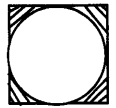# Kerala Syllabus 9th Standard Maths Solutions Chapter 9 Circle Measures

## Kerala State Syllabus 9th Standard Maths Solutions Chapter 9 Circle Measures

### Circle Measures Textual Questions and Answers

Textbook Page No. 131

Circle Measures Class 9 Kerala Syllabus Question 1.
Prove that the circumcentre of an equilateral triangle is the same as its centroid.
i. Calculate the length of a side of an equilateral triangle with vertices on a circle of diameter 1 centimetre.
ii. Calculate the perimeter of such a triangle.
Perpendicular bisector of sides of a triangle that can meet at a point is its circumcenter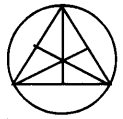Since the triangle is equilateral the perpendicular bisectors of the sides are also median. Since the triangle is equilateral the perpendicular bisectors of the sides are also centroid. That is circumcentre of an equilateral triangle is the same as its centroid.
i. Length of one side of an equilateral triangle is.Circle Measures Class 9 Scert Chapter 9 Kerala Syllabus Question 2.
Calculate the perimeter of a square with vertices on a circle of diameter 1 centimetre.
In the square ABCD
AO = 1/2 cm
In A AEO, ∠EAO = 45°
∠AEO = 90°
Since AO = 1/2 cm
AE = $$\frac{1}{2 \sqrt{2}} \mathrm{cm}$$
(Since Δ AEO is a isosceles right triangle, hypotenuse is √2 times of a perpendicular side.)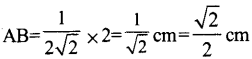Perimeter = $$=4 \times \frac{\sqrt{2}}{2}=2 \sqrt{2} \mathrm{cm}$$

Circle Measures Class 9 Chapter 9 Kerala Syllabus Question 3.
Calculate the perimeter of a regu¬lar hexagon with vertices on a circle of diameter 1 centimetre.
If we draw diagonals through centre of circle inside the regular hexagon it divides the regular hexagon into 6 equilateral triangles.
Let diameter be 1 cm
OA = 1/2 cm
OA = OB = AB.
therefore
One side = 1/2 cm
Perimeter of regular hexagon = 6 × 1/2 = 3 cmTextbook Page No. 134

Hss Live Guru 9th Maths Chapter 9 Kerala Syllabus Question 1.
The perimeter of a regular hexagon with vertices on a circle is 24 centimetres.
i. What is the perimeter of a square with vertices on this circle?
ii What is the perimeter of a square with vertices on a circle of double the diameter?
iii. What is the perimeter of an equilateral triangle with vertices on a circle of diameter half that of the first circle?
Perimeter of a regular hexagon = 24 cm
Length of one side of a regular hexagon = 24/6 = 4 cm
Length of one side of a regular hexagon is equal to the radius of the circle.
i. Diagonal of a square = 8 cm Diagonal of a square is equal to the diameter of the circle.
Let a be the side of the square
a2 + a2 = 82
2a2 = 64
a2 = 64/2 = 32
a= 4√2
∴ One side of a square = 4√2 cm
Perimeter of a square = 4 × 4√2 cm = 16√2 cmii. The perimeter of a square with verti¬ces on a circle of double the diameter 2 × 16√2 = 32 √2 cm
(The perimeters of circles are scaled by the same factor as their diameters.)

iii. One side of an equilateral triangle with vertices on a circle of half the diameter of the first circle
$$=2 \sqrt{2^{2}-1^{2}}=2 \sqrt{3} \mathrm{cm}$$
Perimeter = 3 × 2√3 = 6√3 cm

Circles Class 9 State Syllabus Chapter 9 Kerala Syllabus Question 2.
A wire was bent into a circle of diameter 4 centimetres. What would be the diameter of a circle made by bending a wire of half the length?
The ratio between the perimeters are equal to the ratio between their diameters. The perimeter of the first circle is twice the perimeter of the second circle.
Therefore diameter of the second circle is half of the diameter of the first circle. Diameter of the second circle.
= 4/2 = 2 cm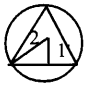Kerala Syllabus 9th Standard Maths Solutions  Question 3.
The perimeter of a circle of diameter 2 metres was measured and found to be about 6.28 metres. How do we compute the perimeter of a circle of diameter 3 metres, without measuring?
If diameter is 2 meters, perimeter is 6.28 meter.
If the diameter is 1 metre, perimeter is 6.28/2 meter.
If the diameter is 3 metres, perimeter = $$\frac{6.28}{2} \times 3=9.42 m$$

Textbook Page No. 137

Hss Live Guru Maths 9 Chapter 9 Kerala Syllabus Question 1.
In the pictures below, a regular hexagon, square and a rectangle are drawn with their vertices on a circle. Calculate the perimeter of each circle.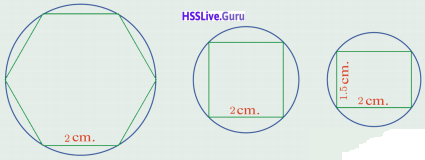a. AB = 2 cm
In the figure triangle are
equilateral triangles,
therefore
Perimeter of circle = 2 πr
= 2 × π × 2 = 4π cm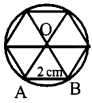b. ABCD is a square
AB = BC = 2 cm, ∠5 = 90°
AC = $$\sqrt{2^{2}+2^{2}}=\sqrt{8}=2 \sqrt{2}$$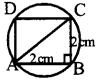Radius of circle = 1/2 × 2√2
= √2 cm
Perimeter of circle = 2π × √2 cm
= 2√2 π cm

c. PR = $$\sqrt{2^{2}+(1.5)^{2}}$$
= $$\sqrt{6.25}=2.5 \mathrm{cm}$$
Radius of circle = 1/2 × 2.5 = 1.25 cm
Perimeter of circle = 2 × π × 1.25
= 2.5 π cm

Kerala Syllabus 9th Standard Maths Notes Question 2.
An isosceles triangle with its vertices on a circle is shown in this picture.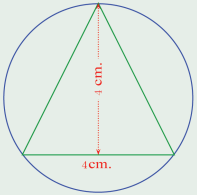What is the perimeter of the circle
Consider the centre of circle as O and triangle as ABC
OC = Radius of circle = r
OD = 4 – r
AO = r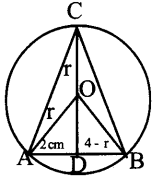Therefore, in triangle AOD
r2=22 + (4 – r)2
r2= 4 +16 – 8r + r2
8r=20;
r = 20/8 = 5/2 = 2.5 cm
∴ Perimeter = 2π × r = 2π × 2.5 cm
= 5π cm

Circles Class 9 Kerala Syllabus Chapter 9 Question 3.
In all the pictures below, the centres of the circles are on the same line. In the first two pictures, the small circles are of the same diameter.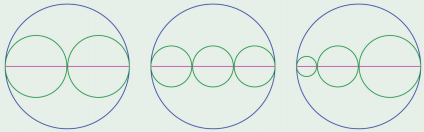Prove that in all pictures, the perimeters of the large circle is the sum of the perimeters of the small circles.
a. Smaller circles have same diameters. Consider the diameter as d, perimeter of smaller circle
= π × diameter = π d
Perimeter of two small circles = 2πd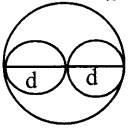Diameter of the large circle = d + d = 2d
Perimeter of the large circle
= π × 2d = 2πd
Therefore perimeters of the large circle is the sum of the perimeters of the small circles.

b. Let d be the diameter of one small circle, then perimeter = π d
Sum of perimeter of three small circles =3 π d
Diameter of the large circle = d + d + d = 3d
Perimeter of the large circle = π × 3d = 3 π d
Therefore perimeters of the large circle is the sum of the perimeters of the small circles.

c. In figure diameter of three circless are different, let consider the diameters of small circles are p, q and r.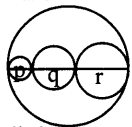Perimeter of first small circle = πp
Perimeter of second small circle = πq
Perimeter of third small circle = πr
Sum of perimeters of three small circles
= πp + πq + πr = π (p + q + r)
Diameter of large circle = p + q + r
Perimeter of large circle = π (p + q + r)
Therefore also here the perimeters of the large circle is the sum of the perimeters of the small circles.

Hss Live Class 9 Maths Chapter 9 Kerala Syllabus Question 4.
In this picture, the circles have the same centre and the line drawn is a diameter of the large circle. How much more is the perimeter of the large circle than the perimeter of the small circle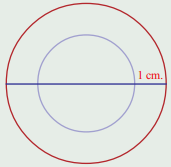If ‘r’ is the radius of the small circle,
radius of the large circle = r + 1
Perimeter of the small circle = 2 π r
Perimeter of the large circle = 2 π (r + 1) = 2 π r + 2 π
i.e., perimeter of the large circle is 2 π units more than the perimeter of the small circle.

Textbook Page No. 141

Hss Live Guru 9 Maths Chapter 9 Kerala Syllabus Question 1.
In the pictures below, find the difference between the areas of the circle and the polygon, up to two decimal places.i. Radius of the small circle = 2cm
Area = π × 22 = 3.14 × 4
= 12.56 cm2
Daigonal of the square = 4 cm
One side of the square = 4/√2 cm
Area of the square = $$\frac{4}{\sqrt{2}} \times \frac{4}{\sqrt{2}}=\frac{16}{2}=8 \mathrm{cm}$$
Differences between the areas = 12.56 – 8 = 4.56 cm

ii. Radius of the circle = 2 cm
Area= π × 22 = 3.14 × 4 = 12.56 cm2
One side of the regular hexagon=2 cm
Area of the regular hexagon = $$6 \times \frac{\sqrt{3} \times 2^{2}}{4}=6 \sqrt{3}$$
= 6 × 1.73 = 10.38 cm2
Differences between the areas = 12.56 – 10.38 = 2.18 cm2

9th Std Kerala Syllabus Maths Solutions Question 2.
The pictures below show circles through the vertices of a square and a rectangleCalculate the areas of the circles
i. One side of a square is 3 cm, therefore its diagonal is 3√2 cm
Diameter of the circle = 3√2 cm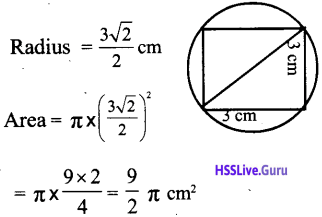ii. Rectangle inside the circle having length 4 cm and breadth 2 cm.
Diagonal = $$\sqrt{4^{2}+2^{2}}=\sqrt{16+4}$$Diameter of the circle = √20 cm
Radius = $$\frac{\sqrt{20}}{2} \mathrm{cm}$$
Area = $$\pi \times\left(\frac{\sqrt{20}}{2}\right)^{2}=\pi \times \frac{20}{4}$$
= 5 π cm2

Hsslive Guru 9th Maths Chapter 9 Kerala Syllabus Question 3.
Draw a square and draw circles cen¬tered on the corners, of radius half the side of the square. Draw another square formed by four of the first square and a circle just fitting into it.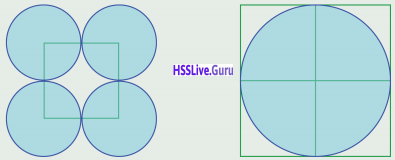Prove that the area of the large circle is equal to the sum of the areas of the four small circles
In the figure length of one side of the square is 2r
Radius of one small circle = r
Perimeter of one small circle = π r2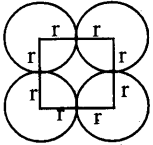Radius of four small circles = 4 π r2
One side of a square in the second figure = 2r + 2r = 4r
Radius of the circle in the figure
= 4r/2 = 2r
perimeter of the circles in the figure = π × (2r)2
= π × 2r × 2r = 4πr2
The area of the large circle is equal to the sum of the areas of the four small circles

Hsslive 9th Maths Chapter 9 Kerala Syllabus Question 4.
In the two pictures below, the squares are of the same size.Prove that the green regions are of the same area.
Let ‘a’ be the side of the square in the picture.
Area of the square = a2
If the four sectors in the vertices are joined together, a circle is formed because the radius of each sector is a/2.
Area of the shaded part = Area of the square – area of the circle.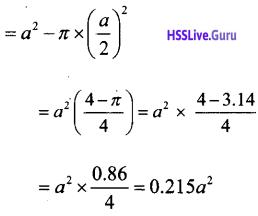ii. In the second picture diameter of the
circle = a Radius = a/2
Area of the shaded part = Area of the square – area of the circle.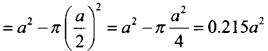i.e., Areas of the shaded portions are equal.

Hsslive Guru 9 Maths Chapter 9 Kerala Syllabus Question 5.
Parts of circles are drawn inside a square as shown in the picture below. Prove that the area of the blue region is half the area of the square.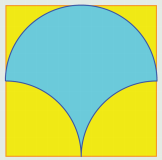Let ‘a’ be the side of the square
Area of the blue part in the half portion of the square is equal to half of the area of the circle having diameter ‘a’.
Area of the blue part in the half portion of the square $$=\frac{1}{2} \times \pi\left(\frac{a}{2}\right)^{2}=\frac{\pi a^{2}}{8}$$
We must subtract area of two circles
having diameter a/2 from the half the
area of the square to get the area of remaining blue shaded part.
Area of remaining blue shaded part.
= $$\frac{a^{2}}{2}-2 \times \frac{1}{4} \times \pi\left(\frac{a}{2}\right)^{2}$$
= $$\frac{\pi a^{2}}{8}+\frac{a^{2}}{2}-\frac{\pi a^{2}}{8}=\frac{a^{2}}{2}$$
i.e., area of the blue part is equal to half the area of the square.

Hss Guru 9 Maths Chapter 9 Kerala Syllabus Question 6.
In the figure, semicircles are drawn with the sides of a right triangle as diameter.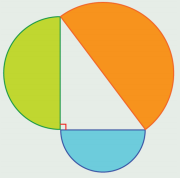Prove that the area of the largest semicircle is the sum of the areas of the smaller ones.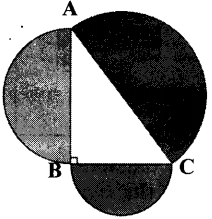In ΔABC, ∠5 = 90°
According to Pythagoras principle,
AB2 + BC2 = AC2 ………. (1)
Radius of the semicircle with diameter
AB = AB/2
Area of the semicircle with diameter AB= $$\frac{1}{2} \times \pi \times \frac{A C^{2}}{4}=\frac{\pi}{8} A C^{2}$$
Sum of the areas of the smaller semicircles = $$\frac{\pi}{8} A B^{2}+\frac{\pi}{8} B C^{2}=\frac{\pi}{8}\left(A B^{2}+B C^{2}\right)$$
= π/8 AC2 = Area of the largest semicircle

Textbook Page No. 148

Hsslive Maths Class 9 Chapter 9 Kerala Syllabus Question 1.
In a circle, the length of an arc of central angle 40° is 3 π centimetres. What is the perimeter of the circle? What is its radius?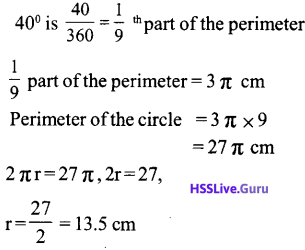Question 2.
In a circle, the length of an arc of central angle 25° is 4 centimetres.
i. In the same circle, what is the length of an arc of central angle 75°?
ii. In a circle of radius one and a half times the radius of this circle, what is the length of an arc of central angle 75°?
i. Length of the arc having central angle 25° = 4 cm
Three times of 25 is 75.
Length of the arc having central angle 75° = 4 x 3 = 12 cm

ii. Length of the arc having central angle 75° and radius r = 12 cm
Length of the arc having central angle 75° and radius 1 1/2 r
= $$12 \times 1 \frac{1}{2}=18 \mathrm{cm}$$

Question 3.
From a bangle of radius 3 centimetres, a piece is to be cut out to make a ring of radius ^ centimetres.
i. What should be the central angle of the piece to be cut out?
ii. The remaining part of the bangle was bent to make a smaller bangle. What is its radius?
Perimeter of the bangle having radius 3 cm = 6 π cm.
Perimeter of the bangle having radius 1/2 cm = π cm.
π is the 1/6 part of 6 π.
Therefore the central angle of the piece to be cut out = 360 × 1/6 = 60°
ii. Length of the remaining part of the bangle = 6π – π = 5π cm
Radius of the other small bangle = 5 π / 2π = 2.5 cm

Question 4.
The picture shows the parts of a circle centred at each vertex of an equilateral triangle and passing through the other two vertices.What is the perimeter of this figure?
Since the triangle is equilateral, each angle is 60°. There are in each side is in a circle of radius 4 cm and the central angle is 60°.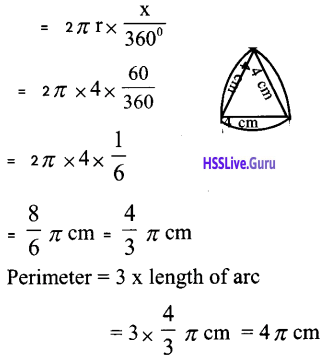Question 5.
Parts of circles are drawn, centred at each vertex of a regular octagon and a figure is cut out as show below: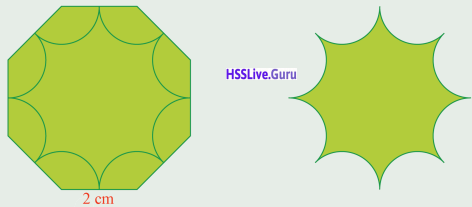Calculate the perimeter of the fig¬ure.
Sum of the angles in an octagon
= (n-2) × 18o° = 6x 180°= 1080° One angle of the regular octagon
= 1080 – 8 = 135°
One side of the regular octagon = 2 cm. Radius if the sectors having centre is each vertices of the circle= 1 cm The second picture shows the cut-down form of 8 sectors having centre angle 135° and radius 1 cm.
The perimeter is found by calculating the length of 8 arc having radius 1 cm and central angle 135°.Textbook Page No. 151

Question 1.
What is the area of a sector of central angle 120° in a circle of radius 3 centimetres? What is the area of a sector of the same central angle in a circle of radius 6 centimetres?
Area of the sector in the circle having radius 3 cm and angle of center 120°
π × 32 × $$\frac { 120 }{ 360 }$$ = 3π cm2
Area of the sector in the circle having radius 6 cm and angle of center 120°
= π × 62 × $$\frac { 120 }{ 360 }$$ = 12π cm2

Question 2.
Calculate the area of the green coloured part of this picture.In the picture area of the shaded part is the difference between the area of the two sectors.
Area of the large sector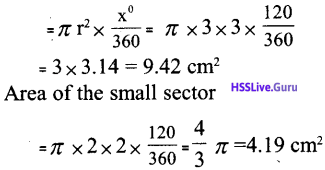Area of the shaded part = 9.42 – 4.19 = 5.23 cm2

Question 3.
Centred at each corner of a regular hexagon, a part of a circle is drawn and a figure is cut out as shown below:What is the area of this figure?
The area of the cut-down portion = Area of the regular hexagon – Area of 6 sectors Area of the regular hexagonArea of the sector = 120°/360° part of area of the circle
(One angle of a regular hexagon is 120°)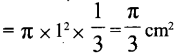Area of the 6 sectors = $$\frac{6 \times \pi}{3}=2 \pi \mathrm{cm}^{2}$$
Area of cut down portion = 6 √3 – 2 π cm2

Question 4.
The picture below shows two circles, each passing through the centre of the other: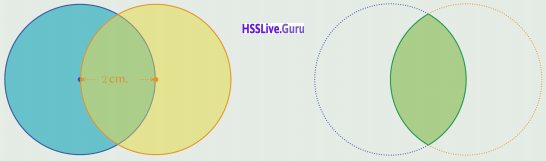Calculate the area of the region common to both.
Consider the picture given below, we can divide the circle into two part by using the line AB, the area of the two portions are same.
That is we get the total area by multi¬plying area of the sector by 2.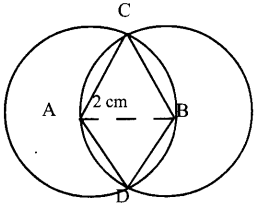We can find out the area of part above the line AB .
Given AB = 2cm
Circles having equal
AC = BC = 2 cm.
AABC is an equilateral triangle so angles are 60° each.
Add the area of sectors having centre A and B .
The area of ΔABC include twice, so we will subtract it once.
Area of sectors having centre A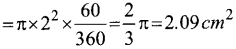Area of sectors having centre BArea of ΔABC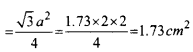Area of the part above the line AB = 2.09 + 2.09 – 1.7 = 2.45 cm2
The area of the region common to both = 2 × 2.45 = 4.90 cm2

Question 5.
The figure shows three circles drawn with their centres on each vertex of an equilateral triangle and passing through the other two ver¬tices;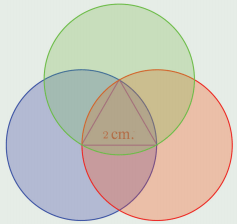Find the area of the region common to all three.
The area of the common part = Area of three sectors – 2 × area of an equilateral triangle having side 2 cm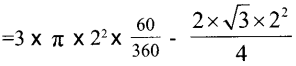= 2 × 3.14 – 2 × 1.73
= 6.28 – 3.46
= 2.82 cm2
The area of the region common to all three = 2.82 cm2

### Circle Measures Exam Oriented Questions And Answers

Question 1.
In the picture PQRS is a square of side 10 cm. A, B, C and D are midpoints of the sides of the squareSemi circles are drawn inside the square.
a. Compute the area of the square.
b. Compute the area of the semi-circle.
c. Compute the area of the shaded part.
a. Since one side of the square is 10 cm,
Area = side x side = 10 × 10= 100 cm2

b. The diameter of one semicircle = half of the side of the square
Diameter = 5 cm
Since the four semicircles are equal. Area of the 4 semicircles
= Area of 2 circles = 2 × πr2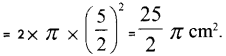c. Area of the shaded part = Area of the square – Area of four semicircles.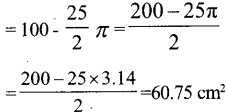Question 2.
If a circular shaped dining table has an area of 31400 cm2, find its radius. What will be its perimeter ?
Area of the table = 31400 cm2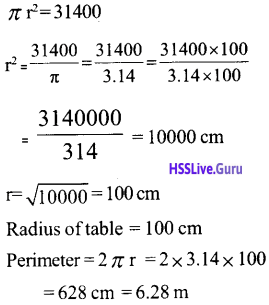Question 3.
In the given picture shown two semicircular shaped iron bar can be cut down from a rectangular shaped iron bar. Calculate the area of the remaining shaded portion.Area of the rectangle = 24 × 14 = 336 cm2
Area of two semicircle = Area of a complete circle
Diameter of the circle = 14 cm
Area of the circle = π r2
= π × 72 =49
π = 153.86 cm2 Area of the remaining portion
= 336 – 153.86= 182.14 cm2

Question 4.
In the figure A, B, C and Dare the points on the square which touches the circle. If the radius of the circle is 6.
a. What is the length of one side of the square?
b. Find the area of the circle.
c. What will be the area of the shaded portion?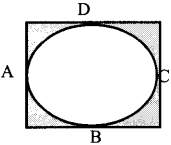a.Diameter of the circle = 6 × 2 = 12 cm
Side of the square = 12 cm
b. Area of the circle = n r2
= n × 6 × 6 = 36n =36 × 3.14 =113.04 cm2
c. Area of the square = 12 × 12 = 144 cm2
Area of the shaded portion = 144 – 113.04 = 30.96 cm2

Question 5.
In the ACB is the arc drawn by taking O as the centre and OA as the radius. Then find the area of the shaded region.The area of sector which has 7 cm radius and 90° central angle =Area of the right angled triangle AOB
$$=\frac{7 \times 7}{2}=24.5$$
Area of the shaded part = 38.455 – 24.5 = 13.965 cm2

Question 6.
In the pictures given below, find the area of the shaded part?’The radius of the sector in the picture (1) is 5cm and its central angle is 40°.
Area of the shaded part $$=\pi \times 5^{2} \times \frac{40}{360}=\frac{25}{9} \pi \mathrm{cm}^{2}$$
The radius of the sector in the picture (2) is 6cm and its central angle is 300° (360 – 60).
Area = $$\pi \times 6^{2} \times \frac{300}{360}=36 \pi \times \frac{5}{6}$$
= 30 π cm2

Question 7.
What amount of reeper is needed to enclose a circular shaped dining table of area 6.28 cm2?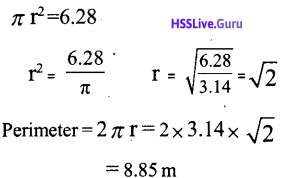Question 8.
A wheel which has 20 cm radius is rotating forward. After 10 rotations what distance will the wheel travel forward?
Radius of the wheel = 20 cm
When the wheel rotates once it will travel the distance same as its area
Perimeter of the wheel = 2 × π × radius = 2 × 3.14 × 20 = 125.64cm
The distance travelled forward when the wheel rotates once = 125.64 cm
The distance travelled forward when the wheel rotates 10 times
= 125.64 × 10 = 1256.4 cm

Question 9.
In the picture the central angles of both the sectors are equal. Sum of the radii of the sectors is 18 cm.
Area of the shaded part is 18 π cm2. Find the radii of the sector.‘Let ‘r’ be the radius of the small sector and ‘R’ be the radius of the large sector.
R + r = 18 …………. (1)
Area of the shaded portion = Area of the large sector – area of the small sector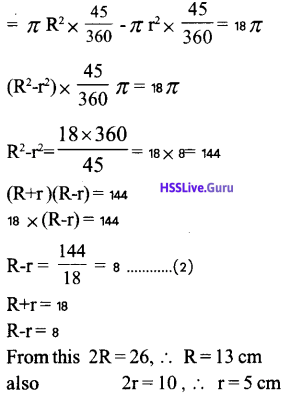Question 10.
In the figure O is the radius of the circle and OABC is a rectangle. OA = 8 cm, OC = 15 cm. Hence find the Area of the shaded portion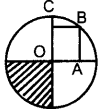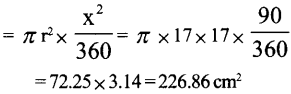Question 11.
The wheel of a vehicle has a diameter 60 cm. For this vehicle travels a distance of 200 m, how many times must this wheel rotates.
‘The distance travelled when the wheel is rotated once =2 π r = 2 × π × 30
= 188.4 cm = 1.884 m.
The time required for the wheel to travel a distance of 200m = 200/1.884 = 106.16 = 106

Question 12.
In the below-given figures, there are two circles with the same centre. Then find the area of the second region.a. Area of the shaded portion =Area of the outer circle – Area of the inner circle
= π R2 – π F= π × 102 – π × 82
= 100π – 64π = 36π
=36 × 3.14 = 113.04 cm2

b. Outer radius = 7 + 2 = 9 cm Inner radius = 7 cm
Area of the shaded portion = π × 92- π × 72 = 81π – 49π = 32π = 32 × 3.14 =100.48 cm2

Inner radius = 10.5 – 1 = 9.5
Area of the shaded portion = π × (10.5)2 – π × (9.5)2
= π × (10.52 – 9.5)2
= π × (10.52 – 9.52)
= π × (10.5 + 9.5) (10.5 – 9.5) = π × 20 × 1 = 62.8 cm2

d. Outer radius = $$\frac { 16π }{ 2π }$$ = 8
Inner radius = $$\frac { 14π }{ 2π }$$ = 7Question 13.
Two semicircular pieces are cut out from a rectangular sheet. Find the area of the remaining portion.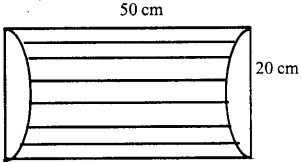Area, of the rectangle = 50 × 20 = 1000 cm2 If the two semicircles cut out are joined it becomes a circle
Its radius = 20/2 = 10 cm
Area of the portion cut out
= πr2 = π × 10 × 10 = 3.14 × 10 × 10 = 314 cm2
Area of the remaining portion
= 1000 – 314 = 686 cm2

Question 14.
If a square, equilateral triangle, regular hexagon and circle have the same perimeter. Which of these has the largest area ?
If a square, equilateral triangle, regular hexagon and circle has a perimeter of 12 cm.
Equilateral triangle
One side = 12/3 = 4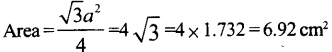Circle has the largest area.

Question 15.
In the figure, if ACB is the arc of circle having O as the centre and OA as the O, radius. Then find the area of the shaded portion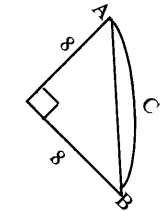Radius of the sector = 8cm Central angle = 90°OBA is a right angled triangle
∴ Area of ΔOBA = 1/2 × 8 × 8 = 32 cm2
Area of shaded portion = 50.24 – 32 = 18.32 cm2

Question 16.
The area of an equilateral triangle is 17300 cm2. Draw circles with radius half the length of one side of the triangle and vertices as the centre of the circle. Calculate the area of the shaded portion.
Area of the equilateral triangleOne side = 200 cm,
Radius of the circle = 100 cm
Central angle of each sector = 60°
Area of one sector = $$\frac{\pi r^{2} \times 60^{\circ}}{360}$$
Area of the three sectors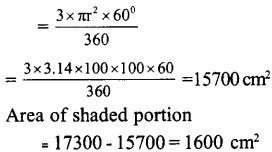Question 17.
In the figure, a side of the regular hexagon has length 20 cm. Find the area of the shaded portion.We have learnt that length of a side of the regular hexagon drawn inside a circle will be equal to the radius of the circle.
So the radius of the circle will be 20 cm.
Area of the circle = πr2 = π × 20 × 20 = 400π = 1256 cm2
Area of the regular hexagon $$=\frac{6 \times \sqrt{3} \times a^{2}}{4}$$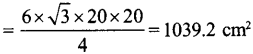Area of shaded portion = 1256 – 1039.2 = 216.8 cm2

Question 18.
If radius of a sector is 7 cm and its perimeter is 2.5 cm. Then find its area.
Area of sector
= 2 × radius + length of the arc = 25
Length of the arc = 25 – 2 × radius = 25 – 14 = 11 cm
Let us check the ratio between the length of the arc and its area
The length of the arc: Area of the sector11 : area of the sector = 2:7
Area of two sectors =7 × 11
Area of the. sector = $$\frac{7 \times 11}{2}$$ = 38.5 cm2

Question 19.
In the figure if the length of one side of a square is 12 cm, then find the area of the shaded portion.